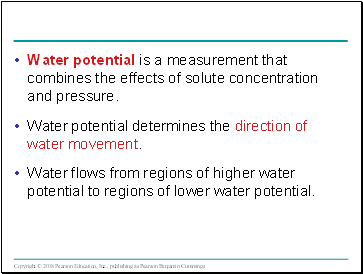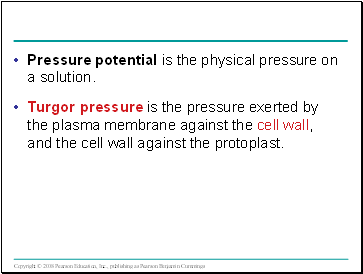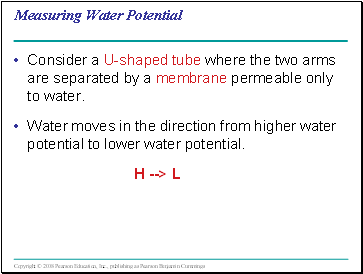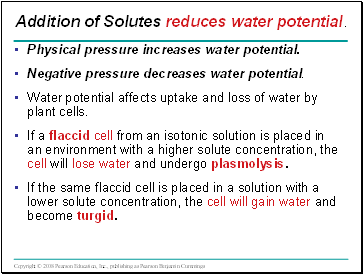# Resource Acquisition and Transport in Vascular PlantsPage 3

#### WATCH ALL SLIDES

Slide 15Water potential is a measurement that combines the effects of solute concentration and pressure.

Water potential determines the direction of water movement.

Water flows from regions of higher water potential to regions of lower water potential.

Slide 16Water potential is measured in units of pressure called megapascals (MPa)

Water potential = 0 MPa for pure water at sea level and room temperature.

Slide 17How Solutes and Pressure Affect Water Potential

Both pressure and solute concentration affect water potential.

The solute potential of a solution is proportional to the number of dissolved molecules (solutes).

Solute potential is also called osmotic potential.

Remember: More solute means less water.

Slide 18Pressure potential is the physical pressure on a solution.

Turgor pressure is the pressure exerted by the plasma membrane against the cell wall, and the cell wall against the protoplast.

Slide 19Measuring Water Potential

Consider a U-shaped tube where the two arms are separated by a membrane permeable only to water.

Water moves in the direction from higher water potential to lower water potential.

H --> L

Slide 20Water potential and water movement.

(a)

(b)

(c)

(d)

0.1 M solution

Positive pressure

Increased positive pressure

Negative pressure (tension)

Pure water

H2O

H2O

H2O

H2O

ψP = 0 ψS = 0

ψP = 0 ψS = −0.23

ψP = 0 ψS = 0

ψP = 0 ψS = 0

ψP = −0.30 ψS = 0

ψP = 0.23 ψS = −0.23

ψP = 0.30 ψS = −0.23

ψP = 0 ψS = −0.23

ψ = 0 MPa

ψ = 0 MPa

ψ = 0 MPa

ψ = 0 MPa

= −0.23 MPa

ψ = 0.07 MPa

ψ = −0.30 MPa

ψ = −0.23 MPa

ψ

Slide 21Physical pressure increases water potential.

Negative pressure decreases water potential.

Water potential affects uptake and loss of water by plant cells.

If a flaccid cell from an isotonic solution is placed in an environment with a higher solute concentration, the cell will lose water and undergo plasmolysis.

If the same flaccid cell is placed in a solution with a lower solute concentration, the cell will gain water and become turgid.

Go to page:
1  2  3  4  5  6  7  8  9  10